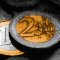Savings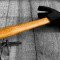DIY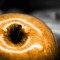Health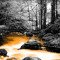EcologyScience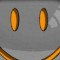Fun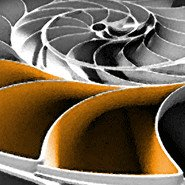# Golden ratio et suite de Fibonacci

Explore the geometric properties of the golden ratio and the Fibonacci sequence, and the relationship between the two. Some particular figures are presented : The golden section, the golden rectangle, the golden spiral, the golden triangle etc.

## Golden ratio

The golden ratio is also called the golden mean or golden section (Latin: sectio aurea). Other names include extreme and mean ratio, medial section, divine proportion, divine section (Latin: sectio divina), golden proportion, golden cut, and golden number.

Mathematicians since Euclid have studied the properties of the golden ratio, including its appearance in the dimensions of a regular pentagon and in a golden rectangle, which may be cut into a square and a smaller rectangle with the same aspect ratio. The golden ratio has also been used to analyze the proportions of natural objects as well as man-made systems such as financial markets, in some cases based on dubious fits to data. The golden ratio appears in some patterns in nature, including the spiral arrangement of leaves and other plant parts.

Some twentieth-century artists and architects, including Le Corbusier and Salvador Dalí, have proportioned their works to approximate the golden ratio—especially in the form of the golden rectangle, in which the ratio of the longer side to the shorter is the golden ratio—believing this proportion to be aesthetically pleasing.

In geometry, the golden ratio is the ratio a / b between two lengths a and b such that: (a + b) / a = a / b. (See "Line segments" below)
The golden ratio is exactly ( 1 + √5 ) / 2, or about 1,618 033 988 7... It's an irrational number, like л (pi), it has no end. For reasons of precision it is the basic formula that is used for the calculations of this page and not a rounded number.

### Line segments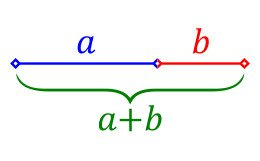Line segments are in the golden ratio if (a + b) / a = a / b; the ratio a / b is then equal to the golden ratio. With this calculation form you can easily calculate line segments in the golden ratio. For example, you have a basic dimension "a" that is 10 and you are looking for b. Just enter the number, click on "calculate" and you get the lower proportion b = 6,180339 ... Conversely, if you know "b" and you are looking for "a" enter the number b and look at the higher proportion.

### Golden rectangle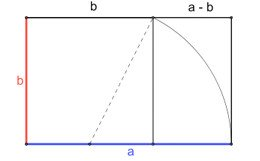The golden rectangle is a rectangle whose length divided by the width is equal to the golden ratio (here also a / b = φ). To build a golden rectangle enter a basic dimension above. If you are looking for the width, enter the length and look "lower proportion", if you are looking for the length enter the width and look at the "higher proportion". We then remove the largest square possible from this rectangle and we obtain a smaller rectangle but with identical proportions. We can also easily build one with a base square and a compass (see opposite).

### Golden spiral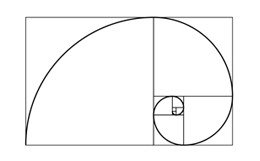If we repeat the previous operation in the small rectangle that has just been created, we obtain again a smaller rectangle with identical proportions, this operation can be repeated at infinity, the rectangles will always be at the same proportions. This figure is the basis of the golden spiral, a spiral that is found much in nature: sunflowers, pine cones, shells, arrangement of leaves or petals on some plants.

### Golden triangle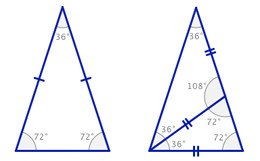The important angles are 108 °; 72 °; 36 °. Every triangle, whatever its dimensions, which has two angles of 72 ° or two of 36 ° is a golden triangle. The ratio of the big side to the small is equal to the golden ratio and you can calculate these dimensions above. In the figure on the right we see that a golden triangle can fit into another. As for the golden rectangle this operation can be repeated to infinity and serves as a basis for the construction of a golden spiral. Golden triangles are also writable in the regular pentagon.

### Regular pentagon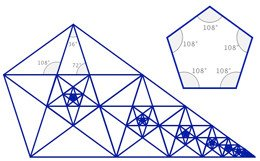This pentagon (5 sides) is inscribed in a circle, all its sides are of the same measures and all its angles are equal (108 °). By inscribing gold triangles we form a smaller pentagon in which we can repeat the operation to infinity. We can thus form infinite and complex repetitive figures that always retain the same proportions. Opposite we can also see that the entire figure itself forms a large golden triangle.

## Fibonacci sequence

The Fibonacci sequence is a sequence of integers in which each term is the sum of the two terms that precede it. It owes its name to Leonardo Fibonacci, an Italian mathematician of the 13th century. This suite was originally part of a study on the growth of a population of rabbits.

### Numbers in the sequence

Number 0 = 0
Number 1 = 1
Number 2 = 0 + 1 = 1
Number 3 = 1 + 1 = 2
Number 4 = 1 + 2 = 3
Number 5 = 2 + 3 = 5
Number 6 = 3 + 5 = 8
Number 7 = 5 + 8 = 13
...
The Fibonnacci sequence is very related to the golden ratio. When dividing a number of the sequence by the preceding number, the result is an estimate of the golden ratio. Conversely, if a number of the sequence is multiplied by the golden ratio, the result is an estimate of the following number in the sequence, and if divided by the golden ratio, the result is an estimate of the preceding number in the sequence. The higher the numbers in the sequence, the closer the link between Fibonacci's sequence and the golden ratio. The numbers 4 and 5 give the golden ratio to the nearest tenth (5/3 = 1.667), the numbers 24 and 25 give it to the billionth (75 025/46 368 = 1 618 033 988 9 ... ).

From the number 40, the link between the golden ratio and the Fibonacci sequence is so close that the system no longer makes the difference. The non-rounded dimension appears as identical to the term. This is a two times (even extremely close).A good approximation of the previous figures can be built with the Fibonacci sequence. One can build a golden rectangle using squares whose sides are equal to the numbers of the Fibonacci sequence. Here we build the golden rectangle only from squares. The squares created will have for successive sides: 1; 1; 2; 3; 5; 8; 13; 21; 34; 55; 89 ...
Fibonacci numbers often appear in nature, as in sunflowers or pine cones. The number of petals of daisy (and other flowers such as sunflower) belongs to the Fibonacci sequence: often 34, 55 or 89. This is explained by the mechanism of development of the plant.

The calculation form on this page is limited to rank 1476 which gives approximately 1.30 x 10308.
To build a golden rectangle with as a base a square of 1 millimeter, the 20th square (the number 20) would be 6,765 m square, the 100th square (number 100) would be more than 37 light years, and 1476 th would be about 1,381 x 10 289 light years away, or 1 followed by 289 zeros. You can check it with this converter